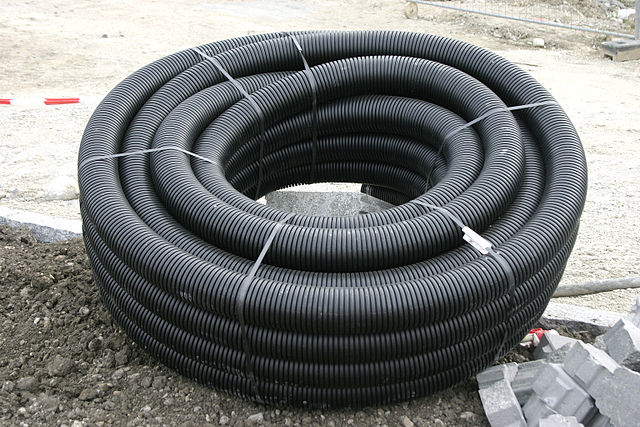# Pipe DreamBob has a pipe with a diameter of $\frac { 6 }{ \sqrt { \pi } }\text{ cm}$ and a length of $3\text{ m}$. How much water could be in this pipe at any one time, in $\text{cm}^3?$

×